# Our first Algebrakit exercise

## Creating a new exercise

Let's create our first Algebrakit exercise to understand how Algebrakit works. You can follow along using the Testbench or the Algebrakit CMS if you have access to it.

We want the student to solve the linear equation $6(p-1)=4p+10$ for $p$. The example below is a live demo of the question we will create, which uses the Multistep question type. Click on the work area to activate it and start solving the equation.

## Authoring component overview

The authoring tool consists of four parts, as seen in the figure below.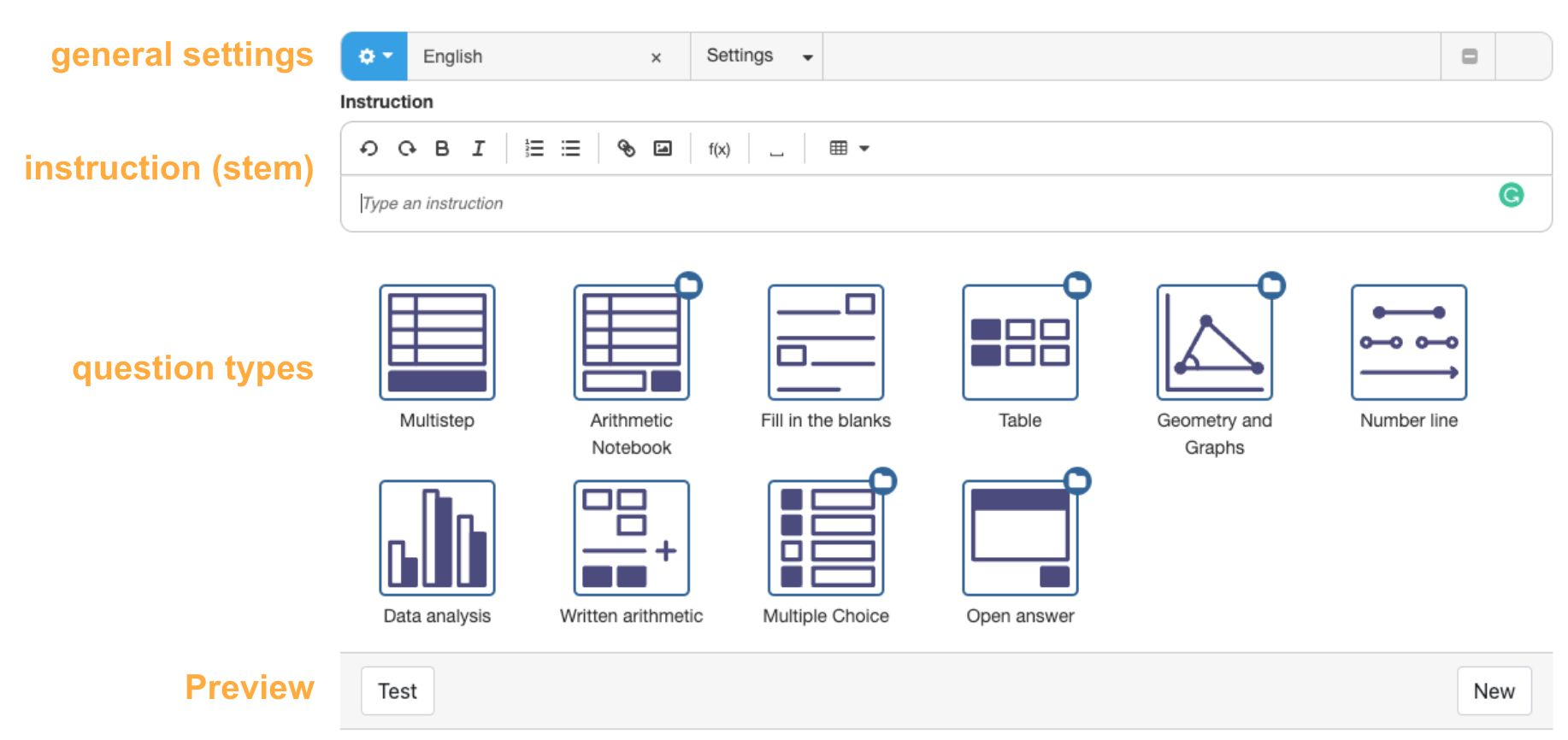Figure: The Authoring tool in Testbench.

• The general settings allow you to configure properties that apply to the exercise. Examples are the student profile to indicate for what kind of student we are creating the problem, or configuring randomness to auto-generate different exercises each time.
• The stem is the instruction for the student. Use the text editor to write your text. There are buttons to add math expressions, images, and tables.
• The question type selector allows you to choose the question type.
• The preview button allows you to test the question you created.

## Creating the stem

Click on the stem editor and type the instruction: 'Solve the equation for p'. You can include the equation as well if you like. For this, click on the formula button $f(x)$ in the editor's menu bar and type $6(p-1)=4p+10$.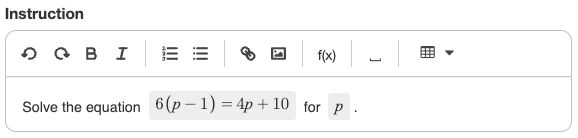Figure: The stem of our exercise.

## Defining the Multistep question type

Click on the Multistep icon to see the input form for this question type. We will configure the equation $6(p-1)=4p+10$ for $p$. We define this through a 'task'.

• Click on the bar below 'Task'.
• In the Task dropdown, select 'Solving equations'.
• Write the equation and the variable in the input fields.

The result should look like this: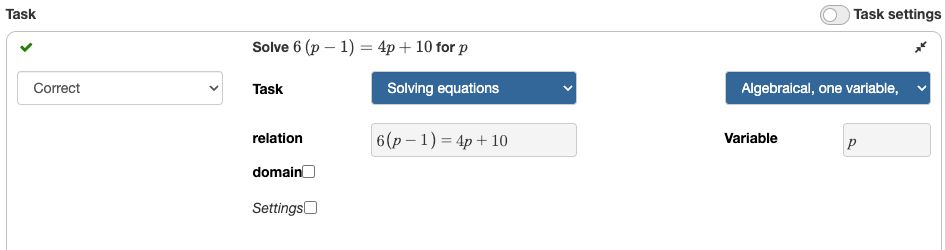Figure: The task definition for the Multistep question

We are ready to run our question!

## Getting a preview

Click on the Test button at the bottom of the screen to preview your exercise.

The first time you run the exercise, Algebrakit will ask you to confirm that $p$ is a variable. You can press the Confirm button.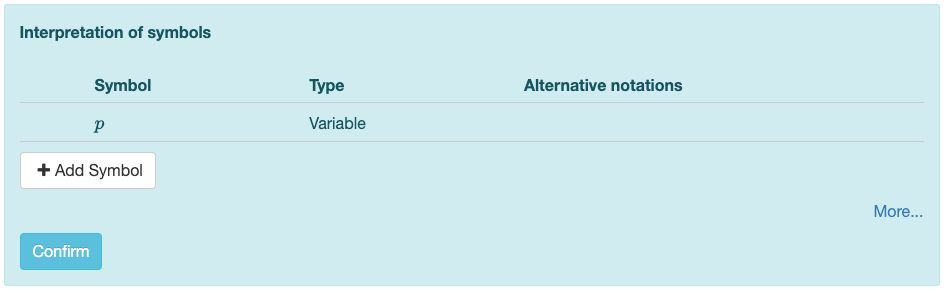Figure: Confirm that $p$ is a variable

The preview shows both the interactive question and the worked solution. The worked solution illustrates how Algebrakit would solve the problem. Click on a step to expand it and see more information.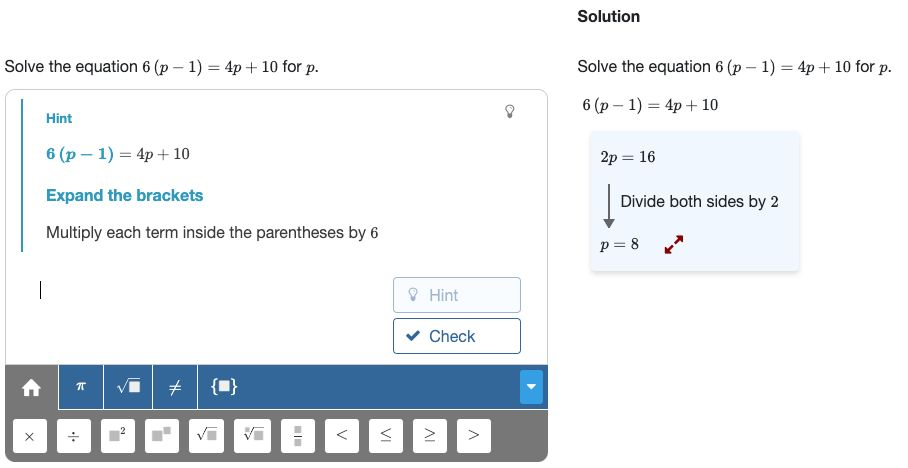Figure: The preview of our exercise.

## Interacting with the exercise

Now let's see what we can do with our new exercise. Activate it by clicking on the input area.

Press the hint button to get a suggestion of what step to take. Note that we didn't enter this hint ourselves; it was generated automatically by Algebrakit.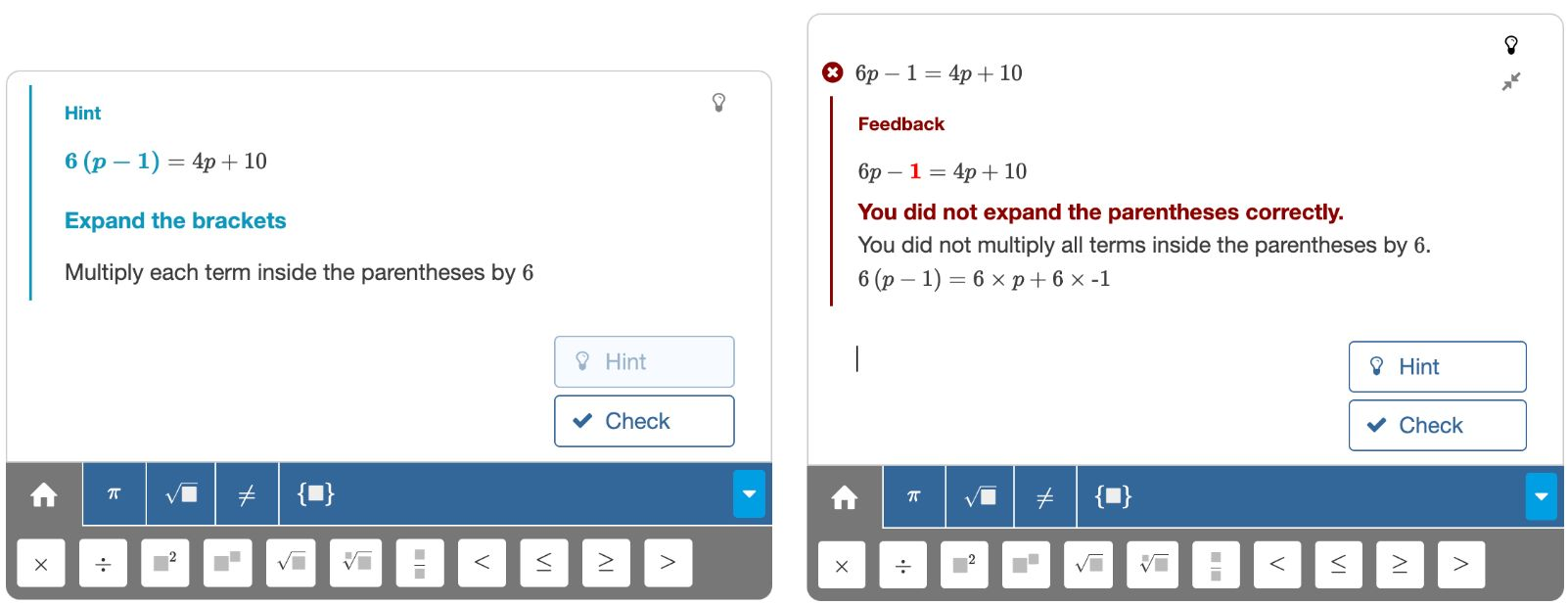Figure: A hint and error feedback generated by Algebrakit.

Now let's input a mistake. Use your keyboard to enter the equation $6p-1=4p+10$ and press enter. Notice how Algebrakit evaluates that the input is incorrect and gives precise feedback on what the mistake was.

Algebrakit uses icons to indicate how it evaluates each input expression.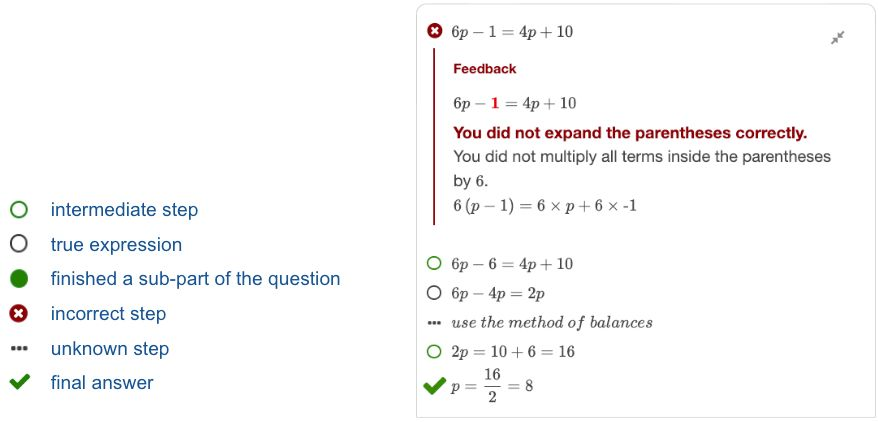Figure: Table of evaluation results.

We invite you to experiment with this question. Try different steps, and see how hints adapt to what you have done. Feel free to neglect Algebrakit's suggestions and choose a different path.

## Randomizing the exercise

With a few steps, we can change this question into a practice question, which is different each time you run it.

Randomization is a powerful feature, and there is much to say about it. We will not go into too much detail here and will show a minimum example.

Open this question in the Testbench and click on the blue settings button in the top bar.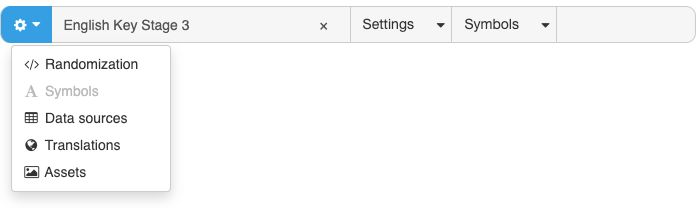Figure: Enabling randomization

Click the Randomization menu item to open the Randomization tab. Here, you can assign random numbers or expressions to exercise parameters. Let's add an exercise parameter that will get a value from -10 to 10, but not zero.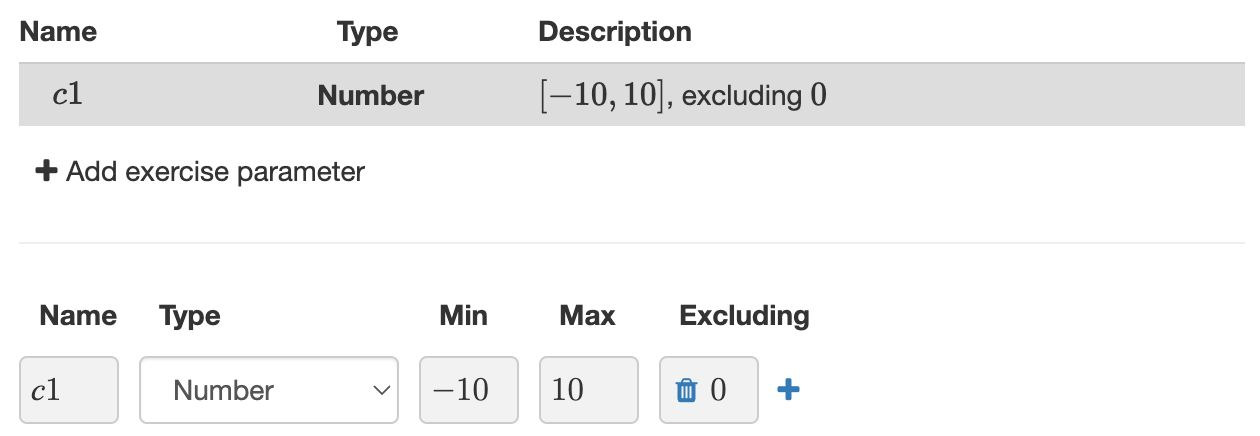Figure: Assigning a random number between -10 and 10 (but not zero) to an exercise parameter

Now let's use that exercise parameter in our equation. In the Task of the Multistep question, replace the '10' with 'c1'. We also want to change the equation in the stem.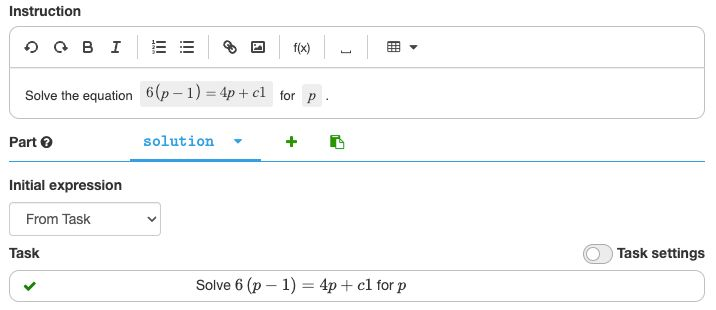Figure: Using the exercise parameter in our question

Now try the question by pressing the Preview button. Press it several times to generate different questions. Each instance will offer hints, error feedback, and a worked solution similar to our initial question.

There are many ways to improve this practice question. For example:

• You can add more exercise parameters to replace all numbers
• You can randomly select different types of linear equations.
• Or you can enforce the linear equations to have integer solutions.

All this is possible and will be explained later in this documentation.